# Inductance Matrix

In Chapter 3, we investigated a tearing mode in a cylindrical tokamak plasma. We found that such a mode resonates with the equilibrium magnetic field at a single magnetic flux-surface, known as the rational surface, whose minor radius,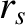, satisfies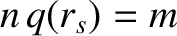, where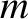and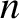are the unique poloidal and toroidal mode numbers, respectively, of the mode. We also found that the mode reconnects magnetic flux at the rational surface, and simultaneously generates a current sheet at the surface that flows parallel to the local magnetic field.

In this chapter, we started to investigate a tearing mode in a toroidal tokamak plasma. We found that such a mode possesses a unique toroidal mode number, but consists of many coupled poloidal harmonics. [See Equations (14.46) and (14.47).] Moreover, the mode resonates at multiple rational surfaces lying within the plasma. The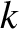th rational surface, minor radius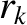, satisfies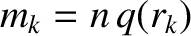, where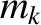is a positive integer. In principle, the mode can reconnect magnetic flux at each rational surface in the plasma, and can also generate a current sheet, flowing parallel to the equilibrium magnetic field, at each surface. We wish to find the relationship between the reconnected magnetic fluxes and the current sheet strengths at the various rational surfaces in the plasma.

Let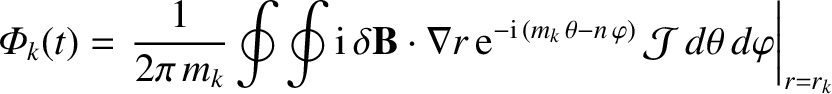(14.89)

be the (complex)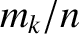helical component of the radial magnetic flux at theth rational surface. It follows from Equations (14.2)–(14.4), (14.44), and (14.65) that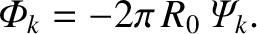(14.90)

Thus,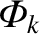is related to the reconnected magnetic flux,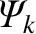(which is actually a flux per unit length), at theth rational surface. Let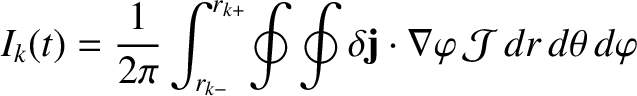(14.91)

be the (complex)helical component of the localized toroidal plasma current flowing at theth rational surface. It follows from Equations (14.2)–(14.4), (14.60), and (14.66) that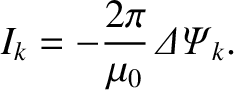(14.92)

Thus,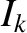is related to the parallel current sheet strength,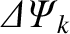, at theth rational surface.

In general, we expect theand theto be related to one another via a complex inductance matrix: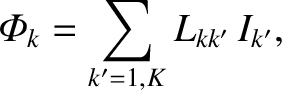(14.93)

for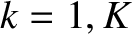. Equations (14.90), (14.92), and (14.93) yield [6,14]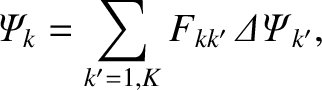(14.94)

where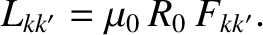(14.95)

Hence, we deduce that the complex matrix,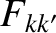, which relates the reconnected fluxes to the current sheet strengths at the various rational surfaces within the plasma, can be regarded as a dimensionless inductance matrix.

The magnetic energy associated with the tearing perturbation is written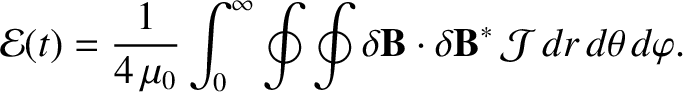(14.96)

Making use of Equations (14.6), (14.44), (14.45), and (14.51)–(14.54), we obtain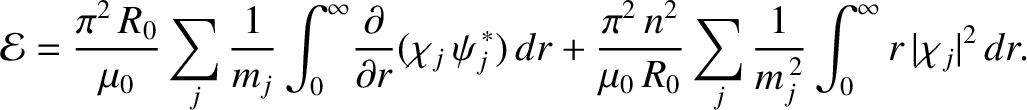(14.97)

The second term on the right-hand side of the previous equation is negligible compared to the first, because we are assuming that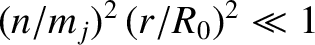. Now, we expect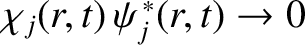as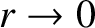. Furthermore, assuming that the plasma is isolated (i.e., it is not interacting with a resistive wall, or an externally generated resonant magnetic perturbation), we also expectas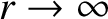. Recalling, however, that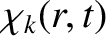is discontinuous across theth rational surface, whereas the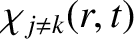and the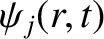are continuous, and making use of Equations (14.65), (14.66), (14.90), and (14.92), we arrive at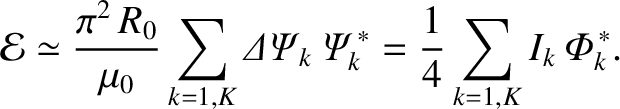(14.98)

Finally, making use of Equation (14.93) and (14.94), we get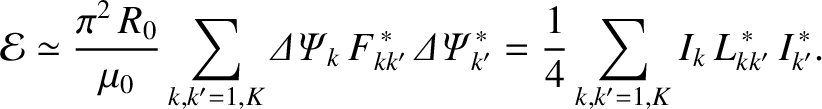(14.99)

Now,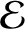is a manifestly real quantity. Hence, it follows from the previous equation that the inductance matrix,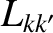, must be Hermitian, which implies that the normalized inductance matrix,, is also Hermitian: that is,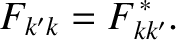(14.100)

There is another reason why the F-matrix,, must be Hermitian. According to Equation (14.70) and (14.94), the net toroidal electromagnetic torque acting on the plasma is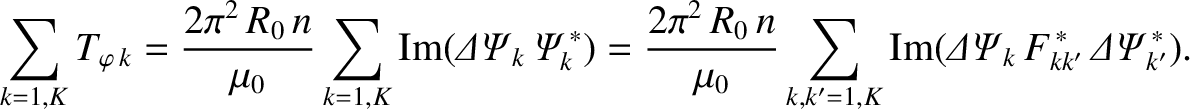(14.101)

However, given that we are assuming that the plasma is isolated, and given that the plasma equilibrium is axisymmetric, this torque must be zero, otherwise toroidal angular momentum would not be conserved . The fact that the F-matrix is Hermitian ensures that the net toroidal torque is indeed zero.